# The Alias Method for weighted sampling

## The Alias Method

The previous technique has excellent best-case behavior, generating a random roll using a single fair die roll and coin flip. On expectation, its worst-case behavior is much worse, though, potentially requiring a linear number of die rolls and coin flips. The reason for this is that, unlike the previous techniques, the algorithm may “miss” and have to repeatedly iterate until it decides on a decision. Graphically, this is because it works by throwing darts at a target that may contain a large amount of empty space not assigned to any outcome. If there were a way to eliminate all of that empty space such that every piece of the target was covered by a rectangle corresponding to some side of the loaded die, then we could just throw a single dart at it and read off the result.

A particularly clever insight we might have is to adjust the height of the rectangle such that instead of having the height match the greatest probability, it matches theaverage probability. Let’s consider our above example. Here, our probabilities are 12,13,112,11212,13,112,112. Since there are four probabilities, the average probability must be 1414. What would happen if we tried normalizing the height of the box to 1414, the average, rather than 1212, the maximum? Let’s see what happens. We begin with rectangles of width 11 whose heights are equal to the initial probabilities: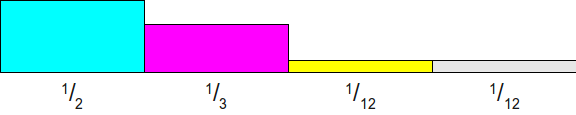Now, we scale all of these rectangles so that a probability of 1414 would have height 1. This works by multiplying each probability by four, yielding this setup:At this point, let’s draw a 1×41×4 rectangle on top of this image. This will represent the target we’ll be shooting at: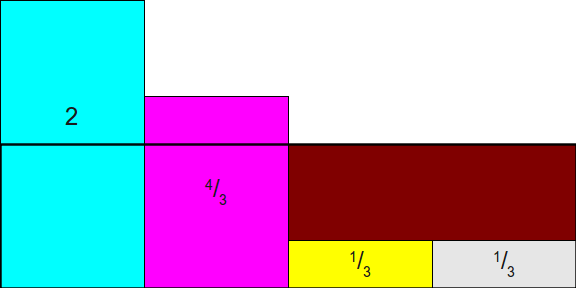As you can see, this doesn’t quite work out, since the rectangles for 1212 and 1313 don’t neatly fit into the box. But what if we allowed ourselves to cut the rectangles into smaller pieces? That is, what if we cut some of the space for the 1212 rectangle off and move it into the empty space above the space for one of the 112112 rectangles? This would give this setup, which still has the vertical bars hanging over, but not by too much:Now, we still have a bit of overhang, but not too much overhang. One way to completely eliminate the overhang would be to move the extra pieces from the 1212 and 1313bars into the empty space, but there’s actually a better solution. Let’s begin by moving enough of the 1212 bar out of the first column and into the third to complete fill in the remaining gap. This will leave a small gap in the first column, but will close the other gap: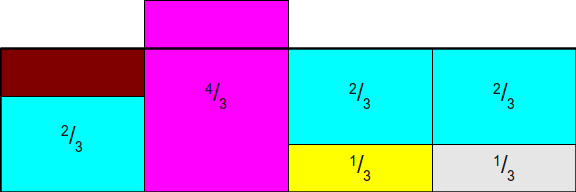Finally, we can tuck the extra overhead from the second column into the first, producing this final rectangle: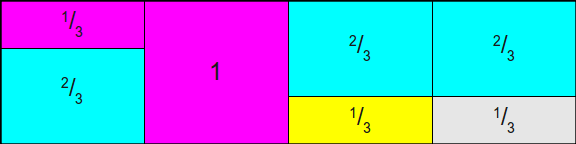What we have below has several excellent properties. First, the total areas of the rectangles representing each side of the loaded die are unchanged from the original; all we’ve done is cut those rectangles into pieces and move them around. This means that as long as the areas of original rectangles are proportionally distributed according to the original probability distribution, the total area dedicated to each side of the die is the same. Second, notice that this new rectangle has no free space in it, meaning that any time we toss a dart at it, we are guaranteed to hit something that will give us an ultimate answer, not empty space that requires another dart toss. This means that a single dart toss suffices to generate our random value. Finally, and most importantly, note that each column has at most two different rectangles in it. This means that we can retain our intuition from before – we roll a die to determine which biased coin to toss, then toss the coin. The difference this time is what the coin toss means. A toss of heads means that we pick one side of the die, and a toss of tails now means that we should pick some other side of the die (rather than rolling again).

At a high level, the alias method works as follows. First, we create rectangles representing each of the different probabilities of the dice sides. Next, we cut those rectangles into pieces and rearrange them so that we completely fill in a rectangular target such that each column has a fixed width and contains rectangles from at most two different sides of the loaded die. Finally, we simulate rolls of the die by tossing darts randomly at the target, which we can do by a combination of a fair die and biased coins.

But how do we even know that it’s possible to cut the original rectangles apart in a way that allows each column to contain at most two different probabilities? This does not seem immediately obvious, but amazingly it’s always possible to do this. Moreover, not only can we cut the rectangles into pieces such that each column contains at most two different rectangles, but we can do so in a way where one of the rectangles in each column is the rectangle initially placed in that column. If you’ll notice, in the above rectangle rearrangement, we always cut a piece of a rectangle and moved it into another column, and never entirely removed a rectangle from its original column. This means that each column in the final arrangement will consist of some rectangle corresponding to the probability initially assigned there, plus (optionally) a second rectangle pulled from some other column. This second rectangle is often called the alias of the column, since the remaining probability of the column is used as an “alias” for some other probability. The use of the term “alias” here gives rise to the name “alias method.”

Before we go into the proof that it’s always possible to distribute the probabilities in this way, we should take a quick second to sketch out how the algorithm actually works. Because each column of the resulting arrangement always contains some (potentially zero-height!) piece of the original rectangle from that column, to store the (potentially) two different rectangles occupying a column, implementations of the alias method typically work by storing two different tables: a probability table ProbProb and an alias table AliasAlias. Both of these tables have size nn. The probability table stores, for each column, the probability within that column that the original rectangle will be chosen, and the alias table stores the identity of the second rectangle (if any) contained in that column. That way, generating a random roll of the die can be done as follows. First, using a fair die, choose some column ii uniformly at random. Next, flip a random coin with probability Prob[i]Prob[i] of coming up heads. If the coin flips heads, output that the die rolled ii, and otherwise output that the die rolled Alias[i]Alias[i]. For example, here are the probability and alias tables for the above configuration:Below is a front-end into a JavaScript implementation of the alias method that has the above probability and alias tables built into it. You can click on the “Generate” button to have it generate a fair die roll and biased coin toss to see what side of the die would be rolled.

## Proving Alias Tables Exist

We now need to formally prove that it is always possible to construct the AliasAlias and ProbProb tables from above. In order to prove that this is always possible, we need to show that it is possible to do the following:

• Construct (npi)×1(n⋅pi)×1 rectangles for each of the probabilities pipi,
• cut them horitzontally into smaller pieces, and
• distribute them into nn columns
• such that each column has height 11,
• no column contains more than two rectangles, and
• some rectangle corresponding to side ii is placed in column ii.

Before going into the proof that it’s always possible to do this, let’s work through an example. Suppose that we have the four probabilities 12,13,112,11212,13,112,112 as above. This is a collection of four probabilities (k=n=4k=n=4) whose sum is 1=441=44. Although we saw above how to fill in the alias table by experimentation, let’s instead try walking through this construction more explicitly by starting with a completely empty table and then filling it in. We begin by scaling all of these probabilities by a factor of four, giving us these probabilities and this empty table:Now, notice that of the four rectangles that we have to distribute, two of them (13,1313,13) are less than 1. This means that they won’t completely fill up a column and will need some other probability to fill in the remainder. Let’s pick one of the two (say, the yellow one) and put it into its appropriate column:Now, we need to somehow make up the difference in the top of the column. To do this, we’ll notice that two of the rectangles that have yet to be distributed have heights greater than 1 (namely, 22 and 4343). Let’s pick one of these two arbitrarily; here, let’s use the 4343. We then distribute enough of the 4343 into the column to fill it completely; this ends up using 2323 of the 4343 up, as shown here:Now, notice what our setup looks like. We now have three rectangles whose total area is 33 and three open columns, so it seems like it should be possible to distribute those rectangles into the three columns. To do so, we’ll use the same intuition as before. Notice that there is at least one rectangle whose height is less than 11, so we’ll pick one arbitrarily (let’s say that we grab the 2323 rectangle) and place it into its column: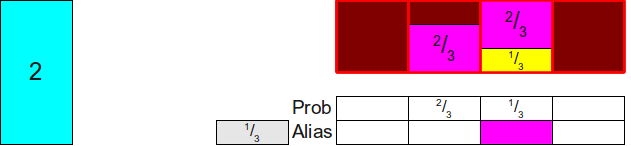We now need to top off the column, so we’ll pick some probability that’s at least 1 and use it to make up the rest of the column. There’s only one choice here (using the 22), so we’ll pull 1313 off of the 22 and put it atop the column: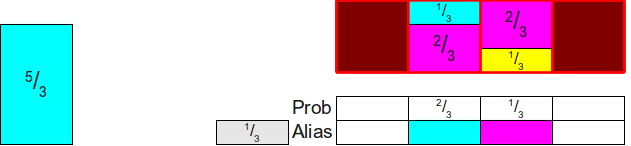And we’re now down to two rectangles shose total area is two. We now repeat this process by finding some rectangle whose height is at most 1 (here, the 1313) and putting it into its column:And then we find some rectangle of height at least 11 to top off the column, using our only choice of the 5353:Now we have just one rectangle remaining, and it has area 1. We can thus finish the construction by just putting that rectangle in its own column:And voilà! We’ve filled in the table.

Notice that the general pattern behind this construction is as follows:

• Find some rectangle that has height at most 1 and place it into its own column, setting the ProbProb table to the height of that rectangle.
• Find some rectangle that has height at least 1 and use it to top off the column, setting the AliasAlias table to correspond to the side of the die represented by the rectangle.

Can we prove that this general construction is always possible? That is, we don’t end up getting “stuck” when distributing probabilities this way? Fortunately, the answer is yes. The intuition behind this is that we’ve scaled all of the probabilities such that the average of the new probabilities is now 1 (because originally it was 1n1n, and we multiplied everything by nn). We know that the minimum of all the scaled probabilities must be no greater than the average and that the maximum of all the scaled probabilities must be no less than the average, so when we first start off there always must be at least one element at most 1 (namely, the smallest of the scaled probabilities) and one element at least one (namely, the largest of the scaled probabilities). We can thus pair these elements together. But what about once we’ve removed these two? Well, when we do this, we end up removing one probability from the total and decreasing the total sum of the scaled probabilities by one. This means that the new average hasn’t changed, since the average scaled probability is one. We can then repeat this procedure over and over again until eventually we’ve paired up all the elements.

We can formalize this argument in the following theorem:

Theorem: Given kk width-one rectangles of heights h0,h1,...,hk1h0,h1,…,hk−1 such that k1i=0hi=k∑i=0k−1hi=k, there is a way of cutting the rectangles and distributing them into kkcolumns, each of which has height 1, such that each column contains at most two different rectangles and the iith column contains at least one piece of the iith rectangle.

Proof: By induction. As a base case, if k=1k=1, then we have just one rectangle and its height must be 1. We can therefore assign it to the 00th column. Thus each column has height 1, contains at most two rectangles, and the 00th column contains at least one piece of the 00th rectangle.

For the inductive step, assume that for some natural number kk the theorem holds and consider any k+1k+1 rectangles of width 11 and heights h0,h1,...,hkh0,h1,…,hk such thatki=0hi=k+1∑i=0khi=k+1. We first claim that there is some height hlhl such that hl1hl≤1 and some different height hghg (such that lgl≠g) such that hg1hg≥1. To see this, assume for the sake of contradiction that there is no hlhl with hl1hl≤1; this would mean that hi>1hi>1 for all natural numbers ii in the range 0ik0≤i≤k. But then we have that k+1=ki=0hi>ki=01=k+1k+1=∑i=0khi>∑i=0k1=k+1, which is clearly impossible. Thus there is some index ll such that hl1hl≤1. Now, suppose for the sake of contradiction that there is no other height hghg (with lgl≠g) such that hg1hg≥1. Then we must have that each other hg<1hg<1, which would (by similar logic) mean that ki=0hi<k+1∑i=0khi<k+1, a contradiction. Consequently, we have that hl1hl≤1 and hg1hg≥1.

Now, consider the following construction. Place hlhl into column ll, and fill the remaining 1hl1−hl space in the llth column with a piece of the rectangle hghg (such space must exist, since 01hl10≤1−hl≤1 and hg1hg≥1). This completely fills the column. We are now left with a collection of kk different pieces of rectangles whose total sum is kk, since we removed 11 total area from the rectangles, whose initial total sum was k+1k+1. Moreover, we have completely filled column ll, so we will never try placing any more pieces of the rectangle there. Thus, by the inductive hypothesis, we can assign the remaining kk rectangles into kk columns while satisfying the above conditions. Combined with the fact that we have now filled column ll, this means that we have a way of filling all the columns while satisfying the constraints. This completes the induction.

This is a constructive proof that says that not only can we always build the alias table, but that the above algorithm of finding a rectangle of height at most one and pairing it with a rectangle of height at least one will always succeed. From here, we can start devising faster and faster algorithms for computing alias tables.

## Generating Alias Tables

Using just what we have said above, we can get a pretty good algorithm for simulating loaded die rolls using the alias method. The initialization works by repeatedly scanning the input probabilities to find a value at most 1 and a value at least 1, combining them together to fill a column:

#### Algorithm: Naive Alias Method

• Initialization:
1. Multiply each probability pipi by nn.
2. Create arrays AliasAlias and ProbProb, each of size nn.
3. For j=1 to n1j=1 to n−1:
1. Find a probability plpl satisfying pl1pl≤1.
2. Find a probability pgpg (with lgl≠g) satisfying pg1pg≥1
3. Set Prob[l]=plProb[l]=pl.
4. Set Alias[l]=gAlias[l]=g.
5. Remove plpl from the list of initial probabilities.
6. Set pg:=pg(1pl)pg:=pg−(1−pl).
4. Let ii be the last probability remaining, which must have weight 1.
5. Set Prob[i]=1Prob[i]=1.
• Generation:
1. Generate a fair die roll from an nn-sided die; call the side ii.
2. Flip a biased coin that comes up heads with probability Prob[i]Prob[i].
3. If the coin comes up “heads,” return ii.
4. Otherwise, return Alias[i]Alias[i].

The generation step of this algorithm is exactly the same as the method described above, and runs in Θ(1)Θ(1). The generation step requires multiple iterations, which are described here. First, we need to spend Θ(n)Θ(n) time scaling each probability by a factor of nn, and need O(n)O(n) time to allocate the two arrays. The inner loop executes Θ(n)Θ(n)times, on each iteration doing O(n)O(n) work to scan the array, remove one of the array elements, and update the probabilities. This gives a total of O(n2)O(n2) total initialization work. If we consider this algorithm in context, we have the following:

Algorithm Initialization Time Generation Time Memory Usage
Best Worst Best Worst Best Worst
Loaded Die from Fair Die Θ(n)Θ(n) O(ni=0di)O(∏i=0ndi) Θ(1)Θ(1) Θ(n)Θ(n) O(ni=0di)O(∏i=0ndi)
Loaded Die from Biased Coins Θ(n)Θ(n) Θ(1)Θ(1) Θ(n)Θ(n) Θ(n)Θ(n)
Roulette Wheel Selection Θ(n)Θ(n) Θ(logn)Θ(log⁡n) Θ(n)Θ(n)
Optimal Roulette Wheel Selection O(n2)O(n2) Θ(1)Θ(1) O(logn)O(log⁡n) Θ(n)Θ(n)
Fair Die/Biased Coin Loaded Die Θ(n)Θ(n) Θ(1)Θ(1) Θ(n)Θ(n)(expected) Θ(n)Θ(n)
Naive Alias Method O(n2)O(n2) Θ(1)Θ(1) Θ(n)Θ(n)

Compared to the other efficient simulation techniques, this naive alias method has a large initialization cost, but can then simulate die rolls extremely efficiently. If we could somehow reduce the initialization cost to something lower (say, O(n)O(n)), then this technique would be strictly better than all of the other techniques employed here.

One simple way to reduce the initialization cost is to use a better data structure for storing the heights as we go. In the naive version, we use an unsorted array to hold all the probabilities, meaning that it takes O(n)O(n) work to locate the two probabilities we want. A better alternative would be to use a balanced binary search tree to hold the values. This way, we could locate the values pgpg and plpl in O(logn)O(log⁡n) time by finding the maximum and minimum values in the tree. Deleting plpl could be done in O(logn)O(log⁡n)time, and updating the probability of pgpg could also be done in O(logn)O(log⁡n) time by simply removing it from the tree and reinserting it. This gives the following algorithm:

#### Algorithm: Alias Method

• Initialization:
1. Create arrays AliasAlias and ProbProb, each of size nn.
2. Create a balanced binary search tree TT.
3. Insert npin⋅pi into TT for each probability ii.
4. For j=1 to n1j=1 to n−1:
1. Find and remove the smallest value in TT; call it plpl.
2. Find and remove the largest value in TT; call it pgpg.
3. Set Prob[l]=plProb[l]=pl.
4. Set Alias[l]=gAlias[l]=g.
5. Set pg:=pg(1pl)pg:=pg−(1−pl).
5. Let ii be the last probability remaining, which must have weight 1.
6. Set Prob[i]=1Prob[i]=1.
• Generation:
1. Generate a fair die roll from an nn-sided die; call the side ii.
2. Flip a biased coin that comes up heads with probability Prob[i]Prob[i].
3. If the coin comes up “heads,” return ii.
4. Otherwise, return Alias[i]Alias[i].

Now, our algorithm’s initialization is much faster. Creating AliasAlias and ProbProb still takes O(n)O(n) time each, and adding the probabilities to the BST TT will take Θ(nlogn)Θ(nlog⁡n) time. From there, we do Θ(n)Θ(n) iterations of filling in the table, each of which takes O(logn)O(log⁡n) work. This gives an overall runtime of O(nlogn)O(nlog⁡n) for the intialization, as seen here:

Algorithm Initialization Time Generation Time Memory Usage
Best Worst Best Worst Best Worst
Loaded Die from Fair Die Θ(n)Θ(n) O(ni=0di)O(∏i=0ndi) Θ(1)Θ(1) Θ(n)Θ(n) O(ni=0di)O(∏i=0ndi)
Loaded Die from Biased Coins Θ(n)Θ(n) Θ(1)Θ(1) Θ(n)Θ(n) Θ(n)Θ(n)
Roulette Wheel Selection Θ(n)Θ(n) Θ(logn)Θ(log⁡n) Θ(n)Θ(n)
Optimal Roulette Wheel Selection O(n2)O(n2) Θ(1)Θ(1) O(logn)O(log⁡n) Θ(n)Θ(n)
Fair Die/Biased Coin Loaded Die Θ(n)Θ(n) Θ(1)Θ(1) Θ(n)Θ(n)(expected) Θ(n)Θ(n)
Naive Alias Method O(n2)O(n2) Θ(1)Θ(1) Θ(n)Θ(n)
Alias Method O(nlogn)O(nlog⁡n) Θ(1)Θ(1) Θ(n)Θ(n)

However, there is an algorithm that runs even faster than this approach. It’s remarkably simple, and is perhaps the cleanest of all of the algorithms for implementing the alias method. This algorithm was originally described in the paper “A Linear Algorithm For Generating Random Numbers With a Given Distribution” by Michael Vose, and has become the standard algorithm for implementing the alias method.

The idea behind Vose’s algorithm is to maintain two worklists, one containing the elements whose height is less than 1 and one containing the elements whose height is at least 1, and to repeatedly pair the first elements of each worklist. On each iteration, we consume the element from the “small” worklist, and potentially move the remainder of the element from the “large” worklist into the “small” worklist. The algorithm maintains several invariants:

• The elements of the “small” worklist are all less than 1.
• The elements of the “large” worklist are all at least 1.
• The sum of the elements in the worklists is always equal to the total number of elements.

For simplicity, each worklist does not store the actual probability, but rather some pointer back to the original probability list saying which side of the loaded die is being referenced. Given these invariants, the algorithm is given below:

#### Caution: This algorithm suffers from numerical inaccuracies. A more numerically sound algorithm is given later.

• Initialization:
1. Create arrays AliasAlias and ProbProb, each of size nn.
2. Create two worklists, SmallSmall and LargeLarge.
3. Multiply each probability by nn.
4. For each scaled probability pipi:
1. If pi<1pi<1, add ii to SmallSmall.
2. Otherwise (pi1pi≥1), add ii to LargeLarge.
5. While SmallSmall is not empty:
1. Remove the first element from SmallSmall; call it ll.
2. Remove the first element from LargeLarge; call it gg.
3. Set Prob[l]=plProb[l]=pl.
4. Set Alias[l]=gAlias[l]=g.
5. Set pg:=pg(1pl)pg:=pg−(1−pl).
6. If pg<1pg<1, add gg to SmallSmall.
7. Otherwise (pg1pg≥1), add gg to LargeLarge.
6. While LargeLarge is not empty:
1. Remove the first element from LargeLarge; call it gg.
2. Set Prob[g]=1Prob[g]=1.
• Generation:
1. Generate a fair die roll from an nn-sided die; call the side ii.
2. Flip a biased coin that comes up heads with probability Prob[i]Prob[i].
3. If the coin comes up “heads,” return ii.
4. Otherwise, return Alias[i]Alias[i].

Given the three above invariants, the first part of this algorithm (everything except the last loop) should be reasonably self-explanatory: we continuously pair some small element from SmallSmall with a large element from LargeLarge as normal, then add the remainder of the large element to the appropriate worklist. The last loop in the algorithm requires some explanation. Once we have exhausted all of the elements from the SmallSmall list, there will be at least one element left over in the LargeLarge list (since if every element was in SmallSmall, the sum of the elements would have to be less than the number of remaining elements, violating the last invariant). Since every element of LargeLargeis at least 1, and since the sum of the kk elements in LargeLarge must be equal to kk, this means that every element in LargeLarge must be exactly equal to 1, since otherwise the total would be too large. This final loop thus sets every large element’s probability to be 1 so that the columns containing the large element are all equal to 1.

In this algorithm, the type of worklist does not matter. Vose’s original paper uses stacks for the worklist because they can be efficiently implemented using arrays, but we could use a queue instead if we’d like. For simplicity, though, we’ll use a stack.

Before doing an analysis of the algorithm, let’s first trace through an example to see how it works. Let’s consider an example of using the seven probabilities 14,15,18,18,110,110,11014,15,18,18,110,110,110. To highlight the fact that the algorithm doesn’t sort the probabilities or require them to be sorted, let’s order them arbitrarily as 18,15,110,14,110,110,1818,15,110,14,110,110,18. The algorithm begins by adding these elements to two work stacks, as shown here:We now place the top of the SmallSmall stack into its slot, moving the magenta rectangle into its final position: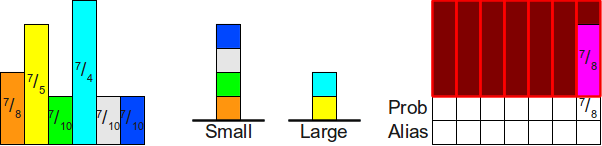Now, we use the top of the LargeLarge stack (the cyan rectangle) to fill in the rest of the column. Since 7418=138174−18=138≥1, we leave the cyan block atop the LargeLarge stack, as shown here:We then repeat this process. We move the rectangle on top of the SmallSmall stack into its column, then top off the difference with the top of the LargeLarge stack:And once more: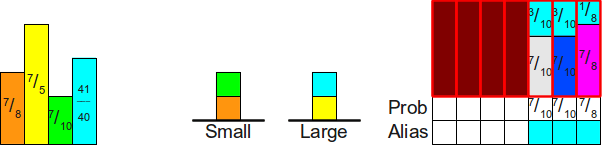When we repeat this process next, we’ll find that while we can use the cyan block to cover the slack space in the table, doing so ends up making the cyan block have height less than one. Consequently, we move the cyan block atop the small stack, as shown here: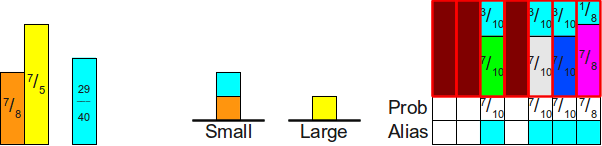Now, when we process the SmallSmall worklist, we end up putting the cyan block in its place, then using the yellow block to fill in the slack:We then process the SmallSmall stack to put the orange block into position, topping it off with the yellow block:And finally, since the SmallSmall stack is empty, we put the yellow block into its own column and are done.We now have a well-formed alias table for these probabilities.

## A Practical Version of Vose’s Algorithm

Unfortunately, the above algorithm, as written, is not numerically stable. On an idealized machine that can do arbitrary-precision real number computations it’s fine, but if you were to try running it using IEEE-754 doubles, it may end up completely failing. There are two sources of inaccuracy that we need to deal with before moving on:

1. The computation to determine whether or not a probability belongs in the SmallSmall or LargeLarge worklist may be inaccurate. Specifically, it may be possible that scaling up the probabilities by a factor of nn has caused probabilities equal to 1n1n to end up being slightly less than 11 (thus ending up in the SmallSmall list rather than the LargeLarge list).
2. The computation that subtracts the appropriate probability mass from a larger probability is not numerically stable and may introduce significant rounding errors. This may end up putting a probability that should be in the LargeLarge list into the SmallSmall list instead.

The combination of these two factors means that we may end up with the algorithm accidentally putting all of the probabilities into the SmallSmall worklist instead of the LargeLargeworklist. As a result, the algorithm may end up failing because it expects the LargeLarge worklist to be nonempty when the SmallSmall worklist is nonempty.

Fortunately, fixing this ends up not being particularly difficult. We will update the inner loop of the algorithm so that it terminates whenever either of the two worklists are empty, so we don’t accidentally end up looking at nonexistent elements from the LargeLarge worklist. Second, when one worklist is empty, we’ll set the remaining probabilities of the elements in the other worklist to all be 11, since, mathematically, this should only occur if all of the remaining probabilites are precisely equal to 11. Finally, we’ll replace the computation that updates the large probabilities with a slightly more stable computation. This is shown here:

#### Algorithm: Vose’s Alias Method

• Initialization:
1. Create arrays AliasAlias and ProbProb, each of size nn.
2. Create two worklists, SmallSmall and LargeLarge.
3. Multiply each probability by nn.
4. For each scaled probability pipi:
1. If pi<1pi<1, add ii to SmallSmall.
2. Otherwise (pi1pi≥1), add ii to LargeLarge.
5. While SmallSmall and LargeLarge are not empty: (LargeLarge might be emptied first)
1. Remove the first element from SmallSmall; call it ll.
2. Remove the first element from LargeLarge; call it gg.
3. Set Prob[l]=plProb[l]=pl.
4. Set Alias[l]=gAlias[l]=g.
5. Set pg:=(pg+pl)1pg:=(pg+pl)−1. (This is a more numerically stable option.)
6. If pg<1pg<1, add gg to SmallSmall.
7. Otherwise (pg1pg≥1), add gg to LargeLarge.
6. While LargeLarge is not empty:
1. Remove the first element from LargeLarge; call it gg.
2. Set Prob[g]=1Prob[g]=1.
7. While SmallSmall is not empty: This is only possible due to numerical instability.
1. Remove the first element from SmallSmall; call it ll.
2. Set Prob[l]=1Prob[l]=1.
• Generation:
1. Generate a fair die roll from an nn-sided die; call the side ii.
2. Flip a biased coin that comes up heads with probability Prob[i]Prob[i].
3. If the coin comes up “heads,” return ii.
4. Otherwise, return Alias[i]Alias[i].

All that’s left to do now is analyze the algorithm’s complexity. Seeding the worklists takes a total of Θ(n)Θ(n) time, because we add each element to exactly one of the worklists. The inner loop does a total of Θ(1)Θ(1) work, since it needs to remove two elements from the worklist, update two arrays, and add one element back to a worklist. It can’t execute more than O(n)O(n) times, since each iteration decreases the number of elements (collectively) in the worklists by one by eliminating the smaller probability. The last two loops can each execute at most O(n)O(n) times, since there are at most O(n)O(n) elements in the LargeLarge and SmallSmall worklists. This gives a total runtime of Θ(n)Θ(n), which (as seen below) is as good as we’re going to get:

Algorithm Initialization Time Generation Time Memory Usage
Best Worst Best Worst Best Worst
Loaded Die from Fair Die Θ(n)Θ(n) O(ni=0di)O(∏i=0ndi) Θ(1)Θ(1) Θ(n)Θ(n) O(ni=0di)O(∏i=0ndi)
Loaded Die from Biased Coins Θ(n)Θ(n) Θ(1)Θ(1) Θ(n)Θ(n) Θ(n)Θ(n)
Roulette Wheel Selection Θ(n)Θ(n) Θ(logn)Θ(log⁡n) Θ(n)Θ(n)
Optimal Roulette Wheel Selection O(n2)O(n2) Θ(1)Θ(1) O(logn)O(log⁡n) Θ(n)Θ(n)
Fair Die/Biased Coin Loaded Die Θ(n)Θ(n) Θ(1)Θ(1) Θ(n)Θ(n)(expected) Θ(n)Θ(n)
Naive Alias Method O(n2)O(n2) Θ(1)Θ(1) Θ(n)Θ(n)
Alias Method O(nlogn)O(nlog⁡n) Θ(1)Θ(1) Θ(n)Θ(n)
Vose’s Alias Method Θ(n)Θ(n) Θ(1)Θ(1) Θ(n)Θ(n)

## Concluding Thoughts

Phew! We’ve covered a lot of ground here! We’ve explored several different methods for simulating loaded dice, beginning with a very simple set of techniques and concluding with extremely fast and efficient algorithms. Each method shows off a different set of techniques, and I find the final version (Vose’s alias method) to be one of the most interesting and elegant algorithms I have ever come across.

If you are interested in seeing code for Vose’s alias method, including a quick summary of what complications arise in practice due to numerical inaccuracy, I have a Java implementation of the alias method available at the Archive of Interesting Code.

If you have any questions, comments, or corrections, please feel free to contact me at [email protected].

Hope this helps!